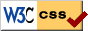# Notes on Diffy Qs - SAGE demonstrations for section 2.6

Press the Evaluate buttons below to launch the SAGE demonstration. After the sage cell is evaluated you should be able to interact with it to change numbers with sliders. You may have to wait a little before the graph appears. Be patient.

## Undamped forced oscillation and resonance

In the following demonstration we graph the solution of

$\frac{x''}{3} + 3x = 10\cos(\omega t)$

You can change the value of $\omega$. Try approaching $\omega = 3$ to see resonance. Also notice the 'beating' behaviour when $\omega$ is not equal to 3. (below $\omega$ is rendered as w).

## Forced oscillation and practical resonance

In the deomnstration below. We graph two solutions of

$x'' + cx' + x = \cos(\omega t)$

One solution starts at rest, that is $x(0) = x'(0) = 0$, and the other starts at $x(0) = x_0$ and $x'(0) = d_0$. Notice how quickly the transient part of the solution goes away and both solutions settle into the steady periodic solution.

We also graph the amplitude $C(\omega)$ for different $\omega$, so that you can find the $\omega$ that gives you the maximum practical resonance. Note how the graph of $C(\omega)$ changes when you change $c$.

(Below, $\omega$ is rendered as w, $x_0$ as x0 and $d_0$ as d0).

The code is mainly due to Ryan Burkhart, it was then slightly modified by Jiří Lebl.